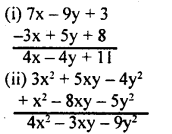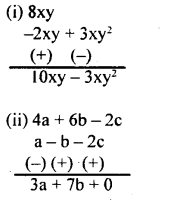# KSEEB Solutions for Class 8 Maths Chapter 2 Algebraic Expressions Ex 2.2

Students can Download Maths Chapter 2 Algebraic Expressions Ex 2.2 Questions and Answers, Notes Pdf, KSEEB Solutions for Class 8 Maths helps you to revise the complete Karnataka State Board Syllabus and score more marks in your examinations.

## Karnataka Board Class 8 Maths Chapter 2 Algebraic Expressions Ex 2.2

Question 1.
Classify into like terms
4x², $$\left(\frac{1}{3} x\right)$$ , – 8x3, xy, 6x3, 4y, -74×3, 8xy, 7 xyz, 3x²
Like terms I= (4x², 3x²)
II =$\left(\frac{1}{3} x\right)$
III = -8x3, 6x3, -74×3
IV = (xy, 8xy)
V = (4y)
VI = (7 xyz)

Question 2.
Simplify
i. 7x – 9y + 3 – 3x + 5y + 8
ii. 3x² + 5xy – 4y² + x² – 8xy – 5y²Question 3.